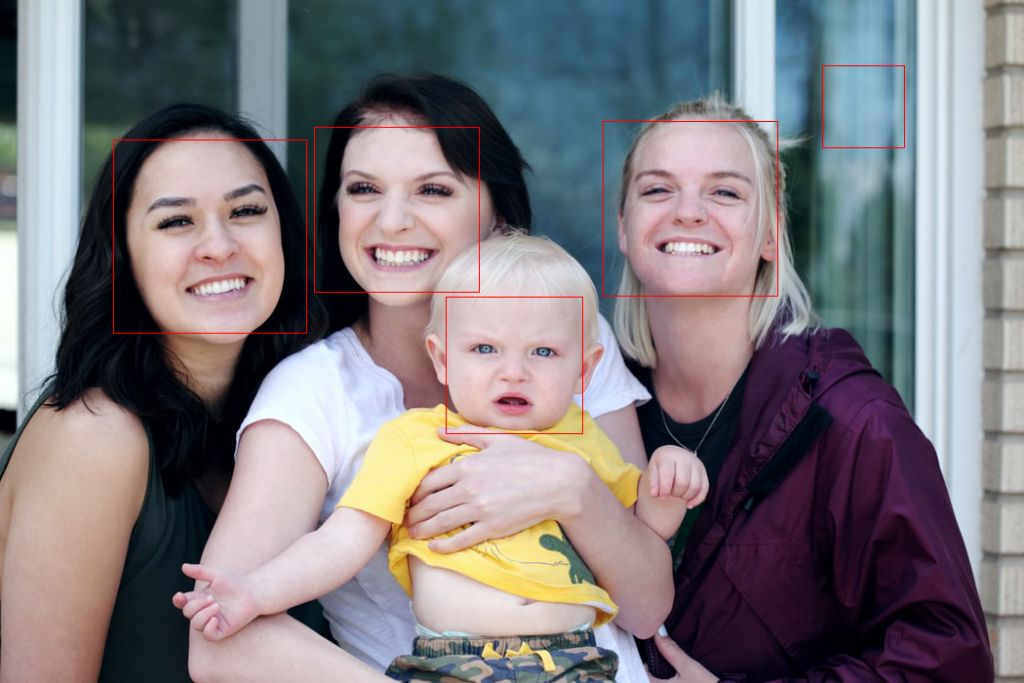# Face Detection in OpenCV

Original article was published by George Pipis on Becoming Human: Artificial Intelligence Magazine#### Example of Face Detection using OpenCV in Python

We will discuss how we can apply Face Detection using OpenCV. We go straightforward with a practical reproducible example.

The logic it the following: We get the image from the URL (or from the hard disk). We convert it to an numpy array and then to a grayscale. Then by applying the proper CascadeClassifier we get the bounding boxes of the faces. Finally, using PIllow (or even OpenCV) we can draw the boxes on the initial image.

`import cv2 as cvimport numpy as npimport PILfrom PIL import Imageimport requestsfrom io import BytesIOfrom PIL import ImageDraw`
`# I have commented out the cat and eye cascade. Notice that the xml files are in the opencv folder that you have downloaded and installed# so it is good a idea to write the whole pathface_cascade = cv.CascadeClassifier('C:\\opencv\\build\\etc\\haarcascades\\haarcascade_frontalface_default.xml')#cat_cascade = cv.CascadeClassifier('C:\\opencv\\build\\etc\\haarcascades\\haarcascade_frontalcatface.xml')#eye_cascade = cv.CascadeClassifier('C:\\opencv\\build\\etc\\haarcascades\\haarcascade_eye.xml')`
`URL = "https://images.unsplash.com/photo-1525267219888-bb077b8792cc?ixlib=rb-1.2.1&ixid=eyJhcHBfaWQiOjEyMDd9&auto=format&fit=crop&w=1050&q=80"response = requests.get(URL)img = Image.open(BytesIO(response.content))img_initial = img.copy()`
`# convert it to np arrayimg = np.asarray(img)`
`gray = cv.cvtColor(img, cv.COLOR_BGR2GRAY)`
`faces = face_cascade.detectMultiScale(gray)# And lets just print those faces out to the screen#print(faces)`
`drawing=ImageDraw.Draw(img_initial)`
`# For each item in faces, lets surround it with a red boxfor x,y,w,h in faces:    # That might be new syntax for you! Recall that faces is a list of rectangles in (x,y,w,h)    # format, that is, a list of lists. Instead of having to do an iteration and then manually    # pull out each item, we can use tuple unpacking to pull out individual items in the sublist    # directly to variables. A really nice python feature    #    # Now we just need to draw our box    drawing.rectangle((x,y,x+w,y+h), outline="red")display(img_initial)`

### Trending AI Articles:

1. Fundamentals of AI, ML and Deep Learning for Product Managers

2. The Unfortunate Power of Deep Learning

3. Graph Neural Network for 3D Object Detection in a Point Cloud

4. Know the biggest Notable difference between AI vs. Machine Learning

And then after drawing the Bounding Boxes we got:

As we can see, we managed to get correctly the four faces BUT we discovered also a “ghost” behind the window…

### Crop the faces to separate images

We can also crop the faces to separate images

`for x,y,w,h in faces:`
`img_initial.crop((x,y,x+w,y+h))    display(img_initial.crop((x,y,x+w,y+h)))`

For example, the first face that we get is:

Notice: In case you wanted to read the image from the hard disk you could simply type the following three lines:

`################################################### read image from the PC##################################################initial_img=Image.open('my_image.jpg')img = cv.imread('my_image.jpg')gray = cv.cvtColor(img, cv.COLOR_BGR2GRAY)##################################################`

### Don’t forget to give us your 👏 !Face Detection in OpenCV was originally published in Becoming Human: Artificial Intelligence Magazine on Medium, where people are continuing the conversation by highlighting and responding to this story.